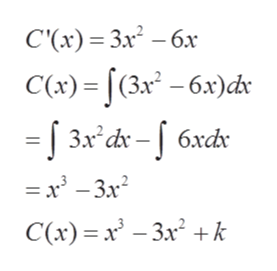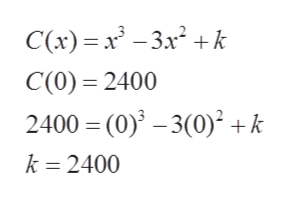Given the marginal cost function C’(x) = 3x2 – 6x, find the cost to produce x items if the fixed costs are \$2400.

Question

Given the marginal cost function C’(x) = 3x2 – 6x, find the cost to produce x items if the fixed costs are \$2400.

Step 1

We are given marginal cost

Step 2

Firstly, we will find antiderivativehelp_outlineImage TranscriptioncloseС() %— Зл3 — бх C() - (3х* - бх)dr Зл* dx - | 6xd — — Зx? С() %3 х - За? +k fullscreen
Step 3

Now, we can find k using initial...help_outlineImage TranscriptioncloseC(x) x3x2k C(0) 2400 2400 (0)-3(0)2 +k k 2400 fullscreen

Want to see the full answer?

See Solution

Want to see this answer and more?

Our solutions are written by experts, many with advanced degrees, and available 24/7

See Solution
Tagged in

Integration